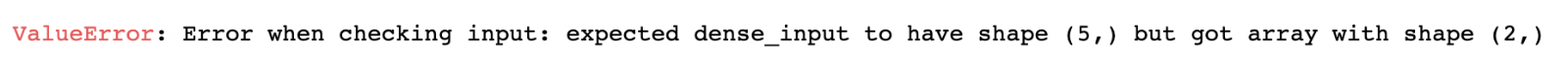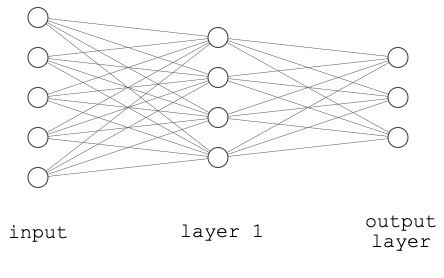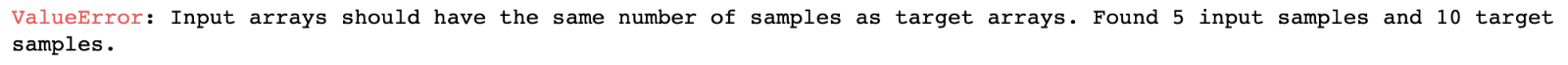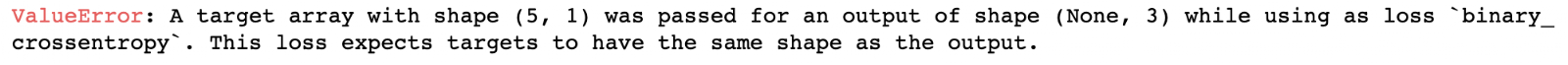How to troubleshoot shape mismatch errors in Keras/TensorFlow

Shape errors in Tensorflow/Keras

As programmers, we all spend a lot of time identifying bugs and fixing them, and new IDEs are making this everyday task easier and easier. Yet debugging Keras/TensorFlow code remains one of the few pains for a machine learning engineer. Based on my own experience, and judging from my students’ questions, a lot of the bugs you will meet are related to shape mismatches in one way or the other, especially when you are building bigger networks with multiple components.

Let’s take a look at how to find such bugs and how to fix them.

Consider the following code for a two-layer shallow neural net.

import numpy as np
from tensorflow.keras.models import Sequential
from tensorflow.keras.layers import Dense

X = np.array([[-0.71400815,  0.57264953],
[-0.17212528, -0.0764972 ],
[ 0.32342135, -0.80562917],
[ 0.13790717, -0.1780438 ],
[ 0.35340332,  0.98442395]])
y = np.array([0, 1, 0, 1, 0, 1, 1, 0, 0, 1])

model = Sequential([
Dense(4, input_shape=(5,), activation='relu'),
Dense(3, activation='sigmoid'),
])

model.compile('sgd',
'binary_crossentropy',
metrics=['accuracy'])

model.fit(X, y)

Looking at our data, we have a dataset X with shape (5,2), i.e. with 5 data points, each described by 2 features. However, whoever wrote the code above defined a network that takes an input with the shape (5,), which is not correct. In fact, running the above code will give:This is not the only kind of shape mismatch error you can encounter when building your model.
For example, a very common set of bugs are those related to the output of the neural net and the loss function used, such as:

All of these error messages are references to the same error: the expected size of a tensor doesn’t match the input/output that you have passed to the model.fit() function call.

You might assume that such long error messages would be helpful at identifying the location of the bug, but they are about as much use as a white crayon.

The input shape in Keras models

The first bugs you should investigate are those that come from the input shape. When building your model, the first layer of the model should contain an input_shape (or input_dim) parameter to specify the dimensions of the input tensors.
According to the Keras docs, the input_shape is a shape tuple (a tuple of integers or None entries, where None indicates that any positive integer may be expected).
In simpler terms:

1. Always define input_shape in the first layer of your model.
2. input_shape is a tuple of integer values defining the dimensions, e.g. (5,6,7,9).
3. Never consider the batch size or the dataset size in the input_shape.

Example:
The following network takes 5 values as input.The input batch shape is (N,5), where N is a positive integer representing the batch size, i.e. the number of data points passed to the model.
When defining our model we must use input_shape = (5,), i.e. only specify the shape of one data point. Here we’re saying that the data point is described by 5 features.

It is generally recommended to use the input_shape parameter instead of input_dim because it can be used for all types of input data (images, sequences …) whereas input_dim can only be used with some 2D layers, such as Dense to specify only one integer (the number of elements in a flat array).

The same example above can be written in two ways:
With input_shape:

Dense(5, input_shape=(5,), ...

With input_dim:

Dense(5, input_dim=5, ...

Notice that with input_shape we must always pass the shape in a tuple with an extra comma: (5,) when our input is flat. (This is Python syntax for tuples with a single element. If we don’t include the trailing comma, Python will ignore the parentheses).

Examples of shape mismatch failure modes

Going back to our initial code, we had an error saying it expected input_shape (5,) and found (2,):Let’s take a look again at our data:

X = np.array([[-0.71400815,  0.57264953],
[-0.17212528, -0.0764972 ],
[ 0.32342135, -0.80562917],
[ 0.13790717, -0.1780438 ],
[ 0.35340332,  0.98442395]])

We can see that its shape is (5,2), where 5 is the batch_size (number of samples) and 2 is the dimension of one input sample, which is the value that we are interested in.
By changing the input_shape to (2, ) we solve this error:

model = Sequential([
Dense(4, input_shape=(2,), activation='relu'),
Dense(3, activation='sigmoid'),
])

Lesson: The input shape is the shape of a single data point and it ignores the batch size.

Our journey is not over yet, because we get another error:

model.compile('sgd',
'binary_crossentropy',
metrics=['accuracy'])
model.fit(X, y)This error is because we have 5 samples in the dataset but 10 labels:

y = np.array([0, 1, 0, 1, 0, 1, 1, 0, 0, 1])

We can fix this problem by making sure that the first dimension in both the input and the output is the same.

y = np.array([0, 1, 0, 1, 0])

Lesson: X and y must have the same number of points, i.e. the same length.

After fixing this bug we get another one:This one comes from the fact that the output of our network has 3 neurons, meaning it outputs three values, whereas our label is a binary label that can be represented with a single output node with values in the interval [0, 1].

This is also a common bug, which can be fixed by changing the number of neurons in the output layer from 3 to 1, as follows:

model = Sequential([
Dense(4, input_shape=(2,), activation='relu'),
Dense(1, activation='sigmoid'),
])

Our neural network now looks like this:

model.compile('sgd',
'binary_crossentropy',
metrics=['accuracy'])
model.fit(X, y)
Train on 5 samples
5/5 [==============================] - 0s 60ms/sample - loss: 0.7099 - acc: 0.6000

And now our code is running perfectly.

import numpy as np
from tensorflow.keras.models import Sequential
from tensorflow.keras.layers import Dense

X = np.array([[-0.71400815,  0.57264953],
[-0.17212528, -0.0764972 ],
[ 0.32342135, -0.80562917],
[ 0.13790717, -0.1780438 ],
[ 0.35340332,  0.98442395]])
y = np.array([0, 1, 0, 1, 0])
model = Sequential([
Dense(4, input_shape=(2,), activation='relu'),
Dense(1, activation='sigmoid'),
])

model.compile('sgd',
'binary_crossentropy',
metrics=['accuracy'])

model.fit(X, y)
Train on 5 samples
5/5 [==============================] - 0s 10ms/sample - loss: 0.8490 - acc: 0.2000

One final common shape mismatch bug is incorrect loss function definition. Most classification tasks are trained using cross-entropy loss (CEL). Keras implements three different CEL definitions:

1. binary_crossentropy: used for binary classification tasks, as the name suggests. This loss treats every output node as an independent output and it typically requires an output sigmoid function, i.e. an output value between 0 and 1.
2. sparse_categorical_crossentropy: used in a multi-class setting where the labels are in the form of label-encoded class. This typically requires a softmax activation function at output.
3. categorical_crossentropy: like the previous loss, it is also used in the multi-class setting, but the labels should be in the form of a one-hot encoded vector, also requiring softmax output.

If you encounter an error related to the output loss such as:

or:

the first thing you should check is the loss function and the kind of encoding you are using for the labels.

Getting the shapes right and building network

Debugging Keras and TensorFlow models can be challenging at best, because you must have both the background needed to understand what is wrong and a set of technical skills that will allow you to fix it.
Instead of spending 80% of your time fixing bugs or reading how-to articles, join us at the Zero to Deep Learning Bootcamp to quickly learn the essentials of deep learning, both theory and practice, allowing you to get things right from the start and save your time and energy.

Tags:Next Article